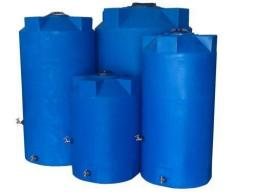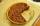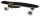# Zdeněk

Zdeněk picked up 15 l of water from a 100-liter full-water barrel. Write a fraction of what part of Zdeněk's water he picked.

Correct result:

f =  0.15

#### Solution:

$f=15\mathrm{/}100=\frac{3}{20}=0.15$We would be very happy if you find an error in the example, spelling mistakes, or inaccuracies, and please send it to us. We thank you!

Showing 1 comment:Shrikari
,I like itTips to related online calculators
Need help calculate sum, simplify or multiply fractions? Try our fraction calculator.

## Next similar math problems:

• Fraction to decimalWrite the fraction 3/22 as a decimal.
• Fraction and a decimalWrite as a fraction and a decimal. One and two plus three and five hundredths
• Mixed2improperWrite the mixed number as an improper fraction. 166 2/3
• Product of two fractionsProduct of two fractions is 9 3/5 . If one of the fraction is 9 3/7. Find the other fraction.
• Lengths of the poolMiguel swam 6 lengths of the pool. Mat swam 3 times as far as Miguel. Lionel swam 1/3 as far as Miguel. How many lengths did mat swim?
• Evaluate expressionCalculate the value of the expression z/3 - 2 z/9 + 1/6, for z = 2
• In fractionsAn ant climbs 2/5 of the pole on the first hour and climbs 1/4 of the pole on the next hour. What part of the pole does the ant climb in two hours?
• Pie IIVili ate three pieces of pie. If it pieces is 1/8 how much pie did he eat?2 and 1 8th plus 1 and 1 3rd =
• Pizza 4Marcus ate half pizza on monday night. He than ate one third of the remaining pizza on Tuesday. Which of the following expressions show how much pizza marcus ate in total?
• CakesOn the bowl were a few cakes. Jane ate one-third of them, Dana ate a quarter of those cakes that remained. a) What part (of the original number of cakes) Dana ate? b) At least how many cakes could be (initially) on thebowl?
• Simple equation 5Solve equation with fractions: X × 3/8 = 1/2
• Pizza 5You have 2/4 of a pizza and you want to share it equally between 2 people how much pizza does each person get?
• Fractions 4How many 2/3s are in 6?
• TeacherTeacher Rem bought 360 pieces of cupcakes for the outreach program of their school. 5/9 of the cupcakes were chocolate flavor and 1/4 wete pandan flavor and the rest were a vanilla flavor. How much more pandan flavor cupcakes than vanilla flavor?
• A storeA store received a shipment of 240 skateboards. In three weeks, it sold 1/3 of those skateboards. How many skateboards did the store sell?
• Equation 20In given equation: 8/9-4/5=2/9+x, find x